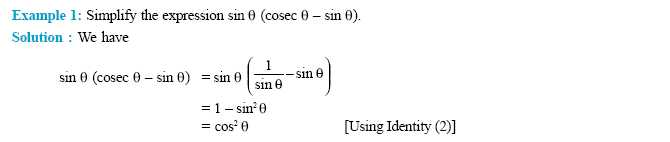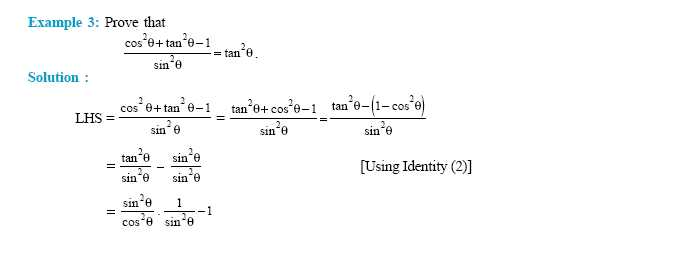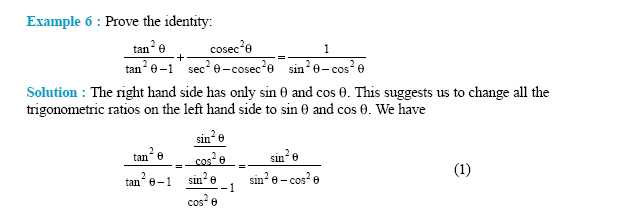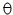Name: ___________________Date:___________________

 Email us to get an instant 20% discount on highly effective K-12 Math & English kwizNET Programs!

High School Mathematics - 210.13 Trignometric Identities - 1Q 1: Show that cot+ tan= sec. cosecfor 00 << 900Answer: Q 2: Show that sec2+ cosec2= sec2cosec2Answer: Q 3: Prove that sec(1 - sin)(sec+ tan) = 1Answer: Q 4: Show that (sin+ cos)2 + (sin- cos)2 = 2Answer: Q 5: Show that tan2+ tan4= sec4- sec2Answer: Q 6: Show that (sin+ cos)2 = 1 + 2 sincosAnswer: Question 7: This question is available to subscribers only! Question 8: This question is available to subscribers only!